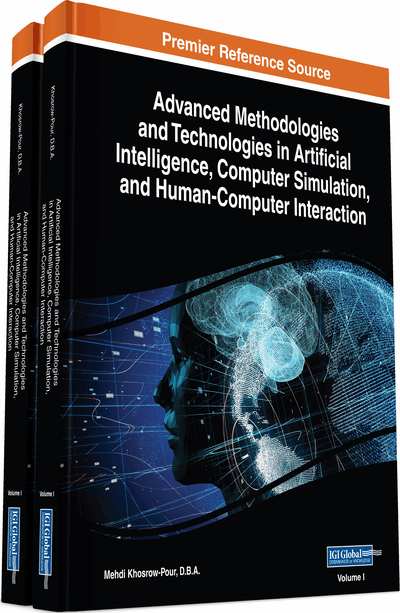# Application of Fuzzy Numbers to Assessment Processes

DOI: 10.4018/978-1-5225-7368-5.ch030
OnDemand:
(Individual Chapters)
Available
\$37.50
No Current Special Offers

## Abstract

A fuzzy number (FN) is a special kind of FS on the set R of real numbers. The four classical arithmetic operations can be defined on FNs, which play an important role in fuzzy mathematics analogous to the role played by the ordinary numbers in crisp mathematics. The simplest form of FNs is the triangular FNs (TFNs), while the trapezoidal FNs (TpFNs) are straightforward generalizations of the TFNs. In the chapter, a combination of the COG defuzzification technique and of the TFNs (or TpFNs) is used as an assessment tool. Examples of assessing student problem-solving abilities and basketball player skills are also presented illustrating in practice the results obtained. This new fuzzy assessment method is validated by comparing its outcomes in the above examples with the corresponding outcomes of two commonly used assessment methods of the traditional logic, the calculation of the mean values, and of the grade point average (GPA) index. Finally, the perspectives of future research on the subject are discussed.
Chapter Preview
Top

## Background

The Fuzzy Numbers (FNs) play an important role in fuzzy mathematics analogous to the role played by the ordinary numbers in crisp mathematics. This section contains the background from the theory of FNs, which is necessary for the purposes of the present work.

### Fuzzy Sets and Logic

The fuzzy sets theory was created in response of expressing mathematically real world situations in which definitions have not clear boundaries. For example, “the high mountains of a country”, “the young people of a city”, “the good players of a team”, etc. The notion of a fuzzy set (FS) was introduced by Zadeh (1965) as follows:

The value mΑ(x) us called the membership degree of x in A. The greater is mΑ(x), the better x satisfies the characteristic property of A. The definition of the membership function is not unique depending on the user’s subjective data, which is usually based on statistical or empirical observations. However, a necessary condition for a FS to give a reliable description of the corresponding real situation is that its membership function’s definition satisfies the common sense. Note that, for reasons of simplicity, many authors identify a FS with its membership function.

A crisp subset A of U can be considered as a FS in U with mΑ(x) = 1, if xA and mΑ(x) = 0, if xA. In this way most properties and operations of crisp sets can be extended to corresponding properties and operations of Fs. For general facts on FS we refer to the book of Klir & Folger (1988).

Fuzzy Logic (FL), based on FS theory, constitutes a generalization and complement of the classical bi-valued logic that finds nowadays many applications to almost all sectors of human activities (e.g. Chapter 6 of Klir & Folger, 1988, Voskoglou, 2011, 2012, 2015 etc.). Due to its nature of characterizing the ambiguous real life situations with multiple values, FL offers, among others, rich resources for assessment purposes, which are more realistic than those of the classical logic (Voskoglou, 2011, 2012, 2015, etc).

The FL approach for a problem’s solution involves the following steps:

• Fuzzification of the problem’s data by representing them with properly defined FSs.

• Evaluation of the fuzzy data by applying principles and methods of FL in order to express the problem’s solution in the form of a unique FS.

• Defuzzification of the problem’s solution in order to “translate” it in our natural language for use with the original real-life problem.

One of the most popular defuzzification methods is the Center of Gravity (COG) technique. When using it, the fuzzy outcomes of the problem’s solution are represented by the coordinates of the COG of the membership’s function graph of the FS involved in the solution (van Broekhoven & De Baets, 2006).

## Complete Chapter List

Search this Book:
Reset